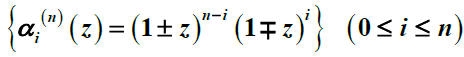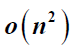# 一类广义Bezout矩阵的研究Study on a Generalized Bezout Matrix

DOI: 10.12677/AAM.2014.32015, PDF, HTML, 下载: 2,488  浏览: 10,452  科研立项经费支持

Abstract: The basesof the polynomial linear spaceare constructed by the bilinear transformation function. Generalized Bezout matrices under two different bases are investigated. By the generating functions of Bezout matrices, a fast algorithm formula and its corresponding triangular decomposition for the elements of this type of Bezout matrix are given. The formula shows that the cost of the algorithm is. Connection between two Bezout matrices under different bases is discussed. Finally, two numerical examples are given to demonstrate the validity of the theory.

  Barnett, S. (1983) Polynomials and linear control system. Marcel Dekker, New York.  Heinig, G. and Rost, K. (1984) Algebraic methods for toeplitz-like matrices and operators. Operator Theory, 13, Birkhauser, Ba-sel.  Barnett, S. and Lancaster, P. (1980) Some properties of the Bezoutian for polynomial matrices. Linear and Multilinear Algebra, 9, 99-110.  Mani, J. and Hartwig, R.E. (1997) Generalized polynomial bases and the Bezoutian. Linear Algebra and Its Applications, 251, 293-320.  Wu, H.Z. (2010) More on polynomial Bezoutians with respect to a general basis. Electronic Journal of Linear Algebra, 21, 154-171.  Yang, Z.H. and Hu, Y.J. (2004) A generalized Bezoutian matrix with respect to a polynomial sequence of interpolatory type. IEEE Transactions on Automatic Control, 49, 1783-1789.  Bini, D.A. and Gemignani, L. (2004) Bernstein-Bezoutian matrices. Theoretical Computer Science, 315, 319-333.  Lancaster, P. and Tismenetsky, M. (1985) The Theory of Matrices. 2nd Edition, Academic Press, London.